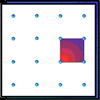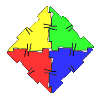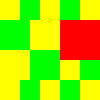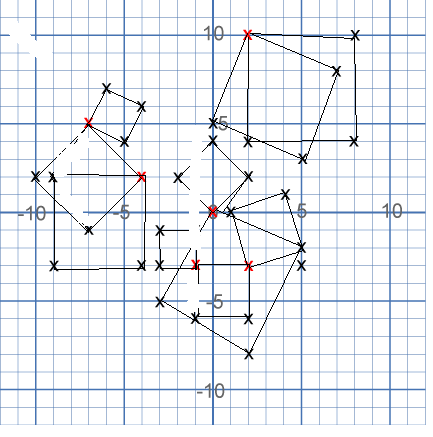#### You may also like### Geoboards

This practical challenge invites you to investigate the different squares you can make on a square geoboard or pegboard.### Polydron

This activity investigates how you might make squares and pentominoes from Polydron.### Tiles on a Patio

How many ways can you find of tiling the square patio, using square tiles of different sizes?

# Ten Hidden Squares

##### Age 7 to 11 Challenge Level:

Harjun sent in this image to show us where the ten squares are:There is just one square which you've mis-drawn a line in I think, Harjun. Can you see which one it is?Akalya and Michelle from Devonshire Primary School and Esther sent in the coordinates of the 10 squares so we can check that way, too:

1.(2,-3) (-1,-3) (-1,-6) (2,-6)
2.(0,0) (5,-3) (2,-8) (-3,-5)
3.(0,0) (2,2) (0,4) (-2,2)
4.(1,0) (4,1) (5,-2) (2,-3)
5.(2,10) (8,10) (8,4) (2,4)
6.(2,10) (7,8) (5,3) (0,5)
7.(-1,-1) (-1,-3) (-3,-3) (-3,-1)
8.(-4,2) (-9,2) (-9,-3) (-4,-3)
9.(-7,5) (-10,2) (-7,-1) (-4,2)
10.(-7,5) (-5,4) (-4,6) (-6,7)

Thank you to all of you - I thought this problem was more difficult than it sounded!